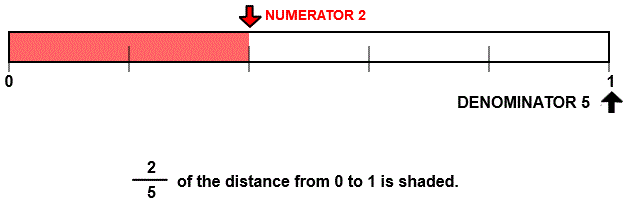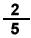# Identify fractions with number line models

## Number line models show the numerator and denominator to give instruction and practice in identifying fractions.

IDENTIFY FRACTIONS
CORRECT:
ATTEMPTS:
SCORE:
PERCENT
NUMERATOR
DENOMINATOR

# IDENTIFY FRACTIONS WITH NUMBER LINE MODELS INSTRUCTIONS

Follow the directions in the dialog box after pressing the <START> button. The <EXPLAIN> button may be pressed to see how to do the example.

Identify Fractions uses number lines to demonstrate the meaning of numerator and denominator.

The following illustration was made by Identify Fractions DesignerThe distance from 0 to 1 is a unit. This unit is divided into 5 equal parts. The arrow tells us that 2 of the parts are chosen. The chosen parts are colored red.

The top number 2 in the numeralis the numerator. The numerator tells us how many parts are chosen.

The bottom number 5 in the numeralis the denominator. The denominator tells us the total number of equal parts into which the unit is divided. In this example there are 5 equal parts in the unit from 0 to 1.

The line between the numerator and denominator is known as the fraction bar. It is also called the division bar.

You will enter the numeralfor the fraction because the arrow points to the 2nd of 5 parts.

For more instruction on identifying fractions go to How To Rename From Mixed Form to Fraction Form.

After you enter the fraction form answer you may press the <REPORT> button. The report will ask for your name but you may submit a code for your name. This report will give same results as on the dialog box. The report may be printed or e-mailed.